# Excel MID function - basic uses and advanced formula examples

MID is one of the Text functions that Microsoft Excel provides for manipulating text strings. At the most basic level, it is used to extract a substring from the middle of the text string. In this tutorial, we will discuss the syntax and specificities of the Excel MID function, and then you will learn a few creative uses to accomplish challenging tasks.

## Excel MID function - syntax and basic uses

Generally speaking, the MID function in Excel is designed to pull a substring from the middle of the original text string. Technically speaking, the MID function returns the specified number of characters starting at the position you specify.

The Excel MID function has the following arguments:

MID(text, start_num, num_chars)

Where:

• Text is the original text string.
• Start_num is the position of the first character that you want to extract.
• Num_chars is the number of characters to extract.

All 3 arguments are required.

For example, to pull 7 characters from the text string in A2, starting with the 8th character, use this formula:

`=MID(A2,8, 7)`

The result might look something similar to this: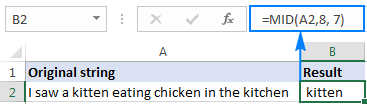### 5 things you should know about Excel MID function

As you have just seen, there's no rocket science in using the MID function in Excel. And remembering the following simple facts will keep you safe from most common errors.

1. The MID function always returns a text string, even if the extracted substring contains only digits. This may be critical if you wish to use the result of your Mid formula within other calculations. To convert an output into a number, use MID in combination with the VALUE function as shown in this example.
2. If start_num is greater than the overall length of the original text, an Excel Mid formula returns an empty string ("").
1. If start_num is less than 1, a Mid formula returns the #VALUE! error.
2. If num_chars is less than 0 (negative number), a Mid formula returns the #VALUE! error. If num_chars is equal to 0, it outputs an empty string (blank cell).
3. If the sum of start_num and num_chars exceeds the total length of the original string, the Excel MID function returns a substring starting from start_num and up to the last character.

## Excel MID function - formula examples

When dealing with real-life tasks in Excel, you will most often need to use MID in combination with other functions as demonstrated in the following examples.

### How to extract first and last name

If you've had a chance to read our recent tutorials, you already know how to pull the first name using the LEFT function and get the last name with the RIGHT function. But as is often the case in Excel, the same thing can be done in a variety of ways.

#### MID formula to get the first name

Assuming the full name is in cell A2, first and last names separated with a space character, you can pull the first name using this formula:

`=MID(A2,1,SEARCH(" ",A2)-1)`

The SEARCH function is used to scan the original string for the space character (" ") and return its position, from which you subtract 1 to avoid trailing spaces. And then, you use the MID function to return a substring beginning with the fist character and up to the character preceding the space, thus fetching the first name.

#### MID formula to get the last name

To extract the last name from A2, use this formula:

`=TRIM(MID(A2,SEARCH(" ",A2),LEN(A2)))`

Again, you use the SEARCH function to determine the starting position (a space). There is no need for us to calculate the end position exactly (as you remember, if start_num and num_chars combined is bigger than the total string length, all remaining characters are returned). So, in the num_chars argument, you simply supply the total length of the original string returned by the LEN function. Instead of LEN, you can put a number that represents the longest surname you expect to find, for example 100. Finally, the TRIM function removes extra spaces, and you get the following result: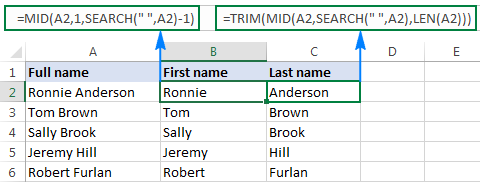### How to get substring between 2 delimiters

Taking the previous example further, if besides first and last names cell A2 also contains a middle name, how do you extract it?

Technically, the task boils down to working out the positions of two spaces in the original string, and you can have it done in this way:

• Like in the previous example, use the SEARCH function to determine the position of the first space (" "), to which you add 1 because you want to start with the character that follows the space. Thus, you get the start_num argument of your Mid formula: SEARCH(" ",A2)+1
• Next, get the position of the 2nd space character by using nested Search functions that instruct Excel to start searching from the 2nd occurrence of the space character: SEARCH(" ",A2,SEARCH(" ",A2)+1)

To find out the number of characters to return, subtract the position of the 1st space from the position of the 2nd space, and then subtract 1 from the result since you don't want any extra spaces in the resulting substring. Thus, you have the num_chars argument: SEARCH (" ", A2, SEARCH (" ",A2)+1) - SEARCH (" ",A2)

With all the arguments put together, here comes the Excel Mid formula to extract a substring between 2 space characters:

=MID(A2, SEARCH(" ",A2)+1, SEARCH (" ", A2, SEARCH (" ",A2)+1) - SEARCH (" ",A2)-1)

The following screenshot shows the result: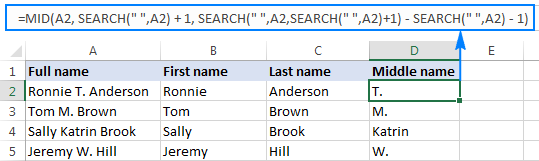In a similar manner, you can extract a substring between any other delimiters:

MID(string, SEARCH(delimiter, string)+1, SEARCH (delimiter, string, SEARCH (delimiter, string)+1) - SEARCH (delimiter, string)-1)

For example, to pull a substring that is separated by a comma and a space, use this formula:

=MID(A2,SEARCH(", ",A2)+1,SEARCH(", ",A2,SEARCH(", ",A2)+1)-SEARCH(", ",A2)-1)

In the following screenshot, this formula is used to extract the state, and it does the job perfectly: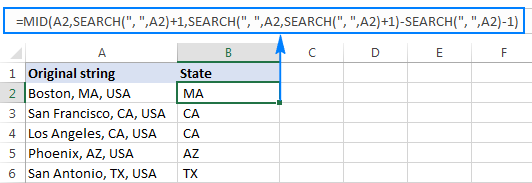### How to extract Nth word from a text string

This example demonstrates an inventive use of a complex Mid formula in Excel, which includes 5 different functions:

• LEN - to get the total string length.
• REPT - repeat a specific character a given number of times.
• SUBSTITUTE - replace one character with another.
• MID - extract a substring.
• TRIM - remove extra spaces.

The generic formula is as follows:

TRIM(MID(SUBSTITUTE(string," ",REPT(" ",LEN(string))), (N-1)*LEN(string)+1, LEN(string)))

Where:

• String is the original text string from which you want to extract the desired word.
• N is the number of word to be extracted.

For instance, to pull the 2nd word from the string in A2, use this formula:

`=TRIM(MID(SUBSTITUTE(A2," ",REPT(" ",LEN(A2))), (2-1)*LEN(A2)+1, LEN(A2)))`

Or, you can input the number of the word to extract (N) in some cell and reference that cell in your formula, like shown in the screenshot below: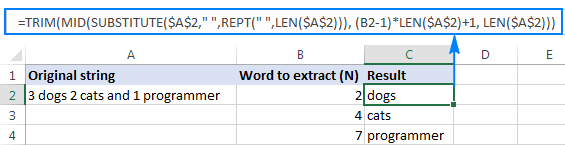#### How this formula works

In essence, the formula wraps each word in the original string with many spaces, finds the desired "spaces-word-spaces" block, extracts it, and then removes extra spaces. To be more specific, the formula works with the following logic:

• The SUBSTITUTE and REPT functions replace each space in the string with multiple spaces. The number of additional spaces is equal to the total length of the original string returned by LEN: SUBSTITUTE(A2," ",REPT(" ",LEN(A2)))

You can think of an intermediate result as of "asteroids" of words drifting in space, like this: spaces-word1-spaces-word2-spaces-word3-… This "spacious" string is supplied to the text argument of our Mid formula.

• Next, you work out the starting position of the substring of interest (start_num argument) using the following equation: (N-1)*LEN(A1)+1. This calculation returns either the position of the first character of the desired word or, more often, the position of some space character in the preceding space separation.
• The number of characters to extract (num_chars argument) is the easiest part - you simply take the overall length of the original string: LEN(A2). At this point, you are left with spaces-desired word-spaces substring.
• Finally, the TRIM function gets rid of leading and trailing spaces.

The above formula works fine in most situations. However, if there happen to be 2 or more consecutive spaces between words, it yields wrong results. To fix this, nest another TRIM function inside SUBSTITUTE to remove excess in-between spaces except for a single space character between words, like this:

`=TRIM(MID(SUBSTITUTE(TRIM(A2)," ",REPT(" ",LEN(A2))), (B2-1)*LEN(A2)+1, LEN(A2)))`

The following screenshot demonstrates the improved formula in action: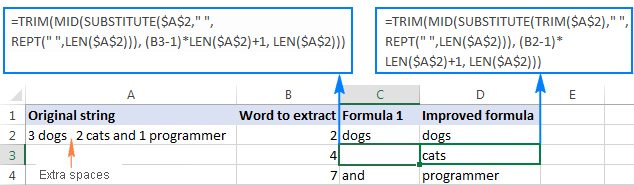If your source strings contain multiple spaces between words as well as very big and very small words, additionally embed a TRIM function into each LEN, just to keep you on the safe side:

`=TRIM(MID(SUBSTITUTE(TRIM(A2)," ",REPT(" ",LEN(TRIM(A2)))), (B2-1)*LEN(TRIM(A2))+1, LEN(TRIM(A2))))`

I agree that this formula looks a bit cumbersome, but it impeccably handles all kinds of strings.

### How to extract a word containing a specific character(s)

This example shows another non-trivial Excel Mid formula that pulls a word containing a specific character(s) from anywhere in the original text string:

TRIM(MID(SUBSTITUTE(string," ",REPT(" ",99)),MAX(1,FIND(char,SUBSTITUTE(string," ",REPT(" ",99)))-50),99))

Assuming the original text is in cell A2, and you are looking to get a substring containing the "\$" character (the price), the formula takes the following shape:

`=TRIM(MID(SUBSTITUTE(A2," ",REPT(" ",99)),MAX(1,FIND("\$",SUBSTITUTE(A2," ",REPT(" ",99)))-50),99))`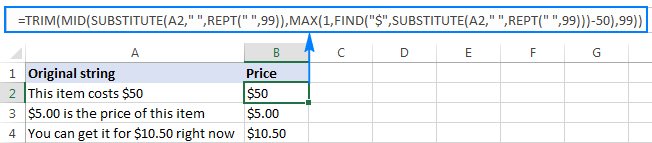In a similar fashion, you can extract email addresses (based on the "@" char), web-site names (based on "www"), and so on.

#### How this formula works

Like in the previous example, the SUBSTITUTE and REPT functions turn every single space in the original text string into multiple spaces, more precisely, 99 spaces.

The FIND function locates the position of the desired character (\$ in this example), from which you subtract 50. This takes you 50 characters back and puts somewhere in the middle of the 99-spaces block that precedes the substring containing the specified character.

The MAX function is used to handle the situation when the desired substring appears in the beginning of the original text string. In this case, the result of FIND()-50 will be a negative number, and MAX(1, FIND()-50) replaces it with 1.

From that starting point, the MID function collects the next 99 characters and returns the substring of interest surrounded by lots of spaces, like this: spaces-substring-spaces.  As usual, the TRIM function helps you eliminate extra spaces.

Tip. If the substring to be extracted is very big, replace 99 and 50 with bigger numbers, say 1000 and 500.

### How to force an Excel Mid formula to return a number

Like other Text functions, Excel MID always returns a text string, even if it contains only digits and looks much like a number. To turn the output into a number, simply "warp" your Mid formula into the VALUE function that converts a text value representing a number to a number.

For example, to extract a 3-char substring beginning with the 7th character and convert it to a number, use this formula:

`=VALUE(MID(A2,7,3))`

The screenshot below demonstrates the result. Please notice the right-aligned numbers pulled into column B, as opposed to the original left-aligning text strings in column A: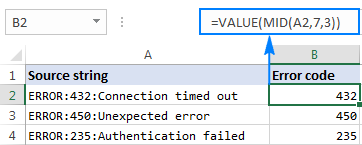The same approach works for more complex formulas as well. In the above example, assuming the error codes are of a variable length, you can extract them using the Mid formula that gets a substring between 2 delimiters, nested within the VALUE function:

`=VALUE(MID(A2,SEARCH(":",A2)+1,SEARCH(":",A2,SEARCH(":",A2)+1)-SEARCH(":",A2)-1))`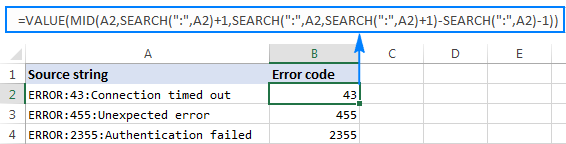This is how you use the MID function in Excel. To better understand the formulas discussed in this tutorial, you are welcome to download a sample Excel MID function workbook. For more Mid formula examples, be sure to check out the following resources. I thank you for reading and hope to see you on our blog next week!

### 52 Responses to "Excel MID function - basic uses and advanced formula examples"

1.Rohan Singh says:

wOW Svetlana!!!! Very Nice Article.

•Svetlana Cheusheva (Ablebits.com Team) says:

•Seeth says:

You are genius!

2.Chuck Allen says:

I'm trying to pull specific digits from a string - WC5-39S-311704-022
I need 6th, 8th & 9-14th, so 9-311704.
Trying for a few days to figure this out.
Any help is appreciated.

•Svetlana Cheusheva (Ablebits.com Team) says:

Hi Chuck,

You can concatenate 3 Mid functions, like this:
=MID(A1,6,1)&MID(A1,8,1)&MID(A1,9,6)

•Mohammed says:

Thank you so much svetlana cheusheva, I am tried to find the above formula tip from many URL's, finally I got it. Thanks my solution solve now! Great help

3.Shashie says:

Hi Svetlana.
I have a column for dates and I want to count how many dates are there? if there are more than one date in a single cell, separated by a comma
(eg.in cell A2=08/02/17,09/02/17,10/02/17). it should come 3.how can i count it using 'countif'formula?
I tried
=countif (a2:a10,"*"&",")
but it doesn't work.
can u help me on this?

•Java_uz says:

=1+LEN(A2)-LEN(SUBSTITUTE(A2,",",""))

4.Java_uz says:

=1+LEN(A2)-LEN(SUBSTITUTE(A2,",",""))

5.mohamad says:

thanks a lot.very very good

6.Netrapal Yadav says:

Awesome thanks a lot..very helpful article.

7.Jensen says:

Hi,

So I have used the MID function for various financial data, but when the data has 1 or 2 zeros as the first decimals, it gives faulty readings (4..2 and "VALUE!" respectively). I assume this has something to do with "NUM_CHARS" and the fact that excel does not recognize a zero at the end. When I use this function: "=MID(40.20,1,LEN(40.20)-3)&"."&MID(40.20,LEN(40.20)-1,2)&"%"" it gives "4..2" rather than "40.20%".

I tried to change the "40.20" to custom rather than "number" but it wouldn't stick. When I went back into format cells, it was back on "number".

If there is a brilliant geek somewhere out there, I would appreciate it sooo much

8.Siddharth says:

HI,

As shown below,i want to divide only period in excel sheet.
is it possible or not, If it is possible could you please let me know.

AKASH ALLAMUNENI 05/01/17-05/31/17 176 HRS 73

9.Chris Levay says:

Hi Svetlana!
Nice info! I have a question. I try to extract some characters from a cell twice. Let me explain:
I have some words I need to eliminate from the original cell. Example:
"My Dog is a beatiful black animal" to become "My Dog is a black animal".
Is there a way to apply the MID function twice in the same cell who let me extract "My Dog is a" and then "black animal"? And if is not possible... Is there a way to do?
Thanks a lot!!!

10.Shelia LeBeauf says:

Hi Svetlana,
This is exactly what I looking for. In the TRIM formula where a dollar amount was extracted, I replaced the "\$" with two characters "V-" and no matter the length of the work (ie: V-1234 or V-1234A), the correct information was extracted. How would you build this in an IF function where the characters being searched vary. Example: T-1234, P-1234, V-1234, C-1234, D-1234, E-1234; IF "T-", IF "P-", IF "V-", so forth.
Thank you for the formula, I just need to build on it. Any help would be greatly appreciated.

•Natalia Sharashova (Ablebits.com Team) says:

Hi, Shelia,

would it be possible for you to send us your workbook with the source data and the result you expect to get to support@ablebits.com? Our technical specialist would take a look into your task to advise you better.
Don't forget to include the link to this comment to your email.

Thanks!

11.Sindre Langberg says:

Hi! Thank you for this very informative article.

Still i have some problems I wonder if you can help me out with

I have this string and many other similar to this:
"1000Miglia 047 7,5x17 5-108 ET45 CB63,4"
And I want to extract the numbers infront of "x" from the second " ".
That means in this text i want to extract the number "7,5"

Is there any simple formula for that?

12.aina says:

I am trying to use the Mid/Find function on this problem but I can't seem to get it correctly.

SNACKNATION 310-845-7744 CA
CANDLEWOOD SUITES ST SOUTHFIELD MI
SUBURBAN IMPORTS OF FARMINGTN HLS MI
EGENFEE* 866-397-2677 WA
KROGER #710 00000071 HARPER WOODS MI

I need to separate these on each column but I'm always getting the #VALUE :(

13.binod says:

want to extract text and numbers from one cell to different rows with function.
each of the following line should be extracted to different rows.
following is in only one cell.
vb61-152262
fg2004-229550
ert2005-065548
df2010-104283
we63-313541
wer2009-100693
r2002-017302
as07-008164

•Gennady Terekhov (Ablebits.com Team) says:

Hello,

1. To extract text:
=SUBSTITUTE(A1,RIGHT(A1,LEN(A1)-MIN(SEARCH({0,1,2,3,4,5,6,7,8,9},A1&"0123456789"))+1),"")

2. To extract numbers:
=RIGHT(A1,LEN(A1)-MIN(SEARCH({0,1,2,3,4,5,6,7,8,9},A1&"0123456789"))+1)

14.Alfredo says:

Thank you Svetlana for the great article! It's awesome!

I would really appreciate some help, I have been going crazy trying to find the solution. Could someone please help me with a formula to extract the name, Barack Obama from the string below?

[{"account_id":555,"name":"Barack Obama "}]

Thank you very much!

•Troy Buschbom says:

=IF(ISNUMBER(SEARCH(E4,C4)),"Found - "&E4,"* No Match *")

to cell D4 and in cell E4 I added the text "Barack Obama"

The formula will look in cell C4 for whatever text you have in cell E4 and if it is found, the cell D4 will reflect what was searched or it will return
* No Match *
A nice feature of SEARCH is that it is not case sensitive.

15.Ahmed says:

What If I Have Like A \$ Sign Followed By Space Then The Number I Want ? Can U Help Me ?

16.Kasun Tharanga says:

Hai,
This is beyond from the subject.
I wants to know how to include (value) for each records which are indicating with minus figures. Eg -50 = (50).

17.Ratu Yufina says:

HI,

As shown below,i want to divide only regional name (mark in ===) in excel sheet.
is it possible or not, If it is possible could you please let me know.

859 - KANINS - KANINS JAKARTA 2 - KANINS JAKARTA 2
===============

Thank You

18.Debbie says:

I have a worksheet with text in column A. I need to find the 2nd time a phrase occurs and extract the number from the right of the common word.
I can extract the entire line, but need the 2 characters from the second occurrence. Can't figure out how to do this.

19.Guna says:

PO=65777570000 ASN=7266271

I need to extract the mid value of the above line that is "65777570000"

•Doug says:

Guna:
A formula to extract 65777570000 uses the MID function.
Using your sample where it is in K6 the formula is:
=MID(K6,4,11) It says, "In K6 go four characters from the left and extract the next 11 characters."

20.Robyn says:

I created a Mid formula to pull out a letter of a number sequence, so I could determine an agency (18N12345 = mid formula pulls out the "N"). However, when I have 18F12345, the F turns red, and the cell color turns pink. The first few times I did it, it crashed excel. Any idea why the "F" has that effect? I have no conditional formats created for these cells.

21.Jasmin U says:

Very useful at work! Thank you very much for making this article. Much appreciated!

22.Chris says:

Hi Anna,

I'm trying to use MID within a SUMPRODUCTS function, but I want to compare the result to a number value, not a string. I tried wrapping the MID array in a VALUE function, but it returns an error. Do you know if there's any way around this?

Essentially my code looks like this:
SUMPRODUCT(A1:A100,--VALUE(MID(B1:B100,3,2))<18)

Thanks

23.Kata says:

Hi, I have this formula: =MID(F3;FIND("09";F3;1);14)

to exract phone number from text string, but it shows #VALUE! when there is no number in the string. I htere a way to insert this funtion into some other to get just an empty cell?

I'm no excel expert so any help is welcome.

THX

•Natalia Sharashova (Ablebits.com Team) says:

Hi, Kata,

You need to wrap your function with IFERROR:
=IFERROR(MID(F3;FIND("09";F3;1);14);"")

Hope this helps!

24.Kata says:

I tryed different things, gor to that function to and it worked until I came across a cell with 6 digit nummer starting with 3XX XXX. I wish I could find VBA code like the one for the extracting e-mail from string, it saved me days of work, unlike this phone number problem.

Function ExtractEmailFun(extractStr As String) As String
'Update 20130829
Dim CharList As String
On Error Resume Next
CheckStr = "[A-Za-z0-9._-]"
OutStr = ""
Index = 1
Do While True
Index1 = VBA.InStr(Index, extractStr, "@")
getStr = ""
If Index1 > 0 Then
For p = Index1 - 1 To 1 Step -1
If Mid(extractStr, p, 1) Like CheckStr Then
getStr = Mid(extractStr, p, 1) & getStr
Else
Exit For
End If
Next
getStr = getStr & "@"
For p = Index1 + 1 To Len(extractStr)
If Mid(extractStr, p, 1) Like CheckStr Then
getStr = getStr & Mid(extractStr, p, 1)
Else
Exit For
End If
Next
Index = Index1 + 1
If OutStr = "" Then
OutStr = getStr
Else
OutStr = OutStr & Chr(10) & getStr
End If
Else
Exit Do
End If
Loop
ExtractEmailFun = OutStr
End Function

•Natalia Sharashova (Ablebits.com Team) says:

Hello, Kata,

We are always ready to help you, but we do not cover the programming area (VBA-related questions).
You may try to find the solution in VBA sections on mrexcel.com or excelforum.com

I wish I could assist you better.

25.Kata says:

Hi,

Thank you! One qestion, is this add in in excel 365 online free?

•Natalia Sharashova (Ablebits.com Team) says:

Hi, Kata,

I'm sorry, but we don't have Text Toolkit for Excel online. The current version works for a desktop Excel only.
You can check all our add-ins for Excel online on this page. These three tools are currently free.

26.Swathi says:

how to extract only the number say suppose there are 100 of rows.. in one row it will be abcd - 2571818 & in second row abcd (173897).

27.RAVI says:

HI I AM RAVI ,
I NEED ONLY 5 WORDS IN THIS FORMAT,CAN ANY ONE HELP ME TO SUPPURATE IN FORMALS BASE,
﻿S5235/ACHYUTH

•Ragavendran says:

Dear Mr Ravi
Please use this formula to find the 5 charecter in specified cell.

=MID(A1,FIND("S",A1,1),5)
Note : A1 is the example cell reference for your requirement.

28.Lavish Angel G says:

Hi there, I need help.
Lets say I have a series starting with A until V
Lets say I have a sequence that reads "ABCDEFGHIJK", now I need something that can say TRUE when my series contains more than 7 letters from the group A to K, and false when my series contains less than 7 letters from the group A to K.

For example.
ABCDEFGHIJK = TRUE
ABCDEFGLMNO = FALSE
ABCDELMNOPQ = FALSE
ABCDEFGHRST = TRUE

Any help would be greatly appreciated. :)

29.Gregory Williams says:

Hello,
I am looking to rearrange a date using Left, Mid and Right; unless someone has a better way to do this. Mt date is for example 20190101 ... I want to reformat the call to look like 01/01/2019. I am trying =RIGHT(A1,2),MID(A1,4,2),LEFT(A1,4)

•Gregory Williams says:

I don't know how to delete my comment but as I was about to give up I figured it out!
=MID(A1,5,2)&"/"&RIGHT(A1,2)&"/"&LEFT(A1,4)
20190101 changes to 01/01/2019

30.Charlie says:

Hi, Fantastic Article!
Is there any way to search for additional data within the same cell and have all the data that is pulled out, separated by a comma?
I have a cell with 1 to 5 user names and ID's. The ID's are in parenthesis and I want to pull the ID's out to be separated by a comma.
My worksheet is setup like the data below in a single column, but with 240 rows.
Smith,Jim A (123456)Doe,John (789123)
Doe,Jane(393027)
Boss,Tim (293029)Deer,Fred(001289)Stern,Greg(148900)
I'm trying to get the data to return like this:
123456,789123
393027
293029,001289,148900

31.Leandra says:

trying to get it to use an IF function along with a MID in extracting numbers withing a string of both text and numbers.

PBQ4X5 - using =MID(A2, SEARCH("Q",A2)+1, 1) to return the "4" and the same with "X" to return the number associated with it. Unfortunately when it runs across a sequence that doesn't include either the X or the Q (PBI5G3), I get "#VALUE!". I also get "#VALUE when trying to "sum" the number columns that the formula returns. Trying to come up with an IF formula to incorporate with the MID and SEARCH so that it will return a "0" when it can't find the particular text (Q or X). Any help would be appreciated, I've been working on this for quite a while.

32.SOLOMON says:

HI, I used this formula =TRIM(MID(SUBSTITUTE(E4991," ",REPT(" ",99)),MAX(1,FIND("IL",SUBSTITUTE(E4991," ",REPT(" ",99)))-50),99)), which worked well with my case 2 and 3 below but did not give the desired result for case 1 and 4 due to the fact that there are no space in the case 1.
Result i need is 16 characters starting with IL included.
Case 1 : MF20020011111IL11000NG0000001
case 2 : MF20020022222 IL11000NG0000002 revalued
case 3 : IL11000NG0000002 MF20020022222 revalued
Case 4 : MF20020033333IL11000NG0000003revalued
Thanks

33.Munther says:

Can i make mid function start from the right direction and select the number on the left side of the spesfic number with out use right function ?

34.Naneida says:

Hi!
I am using the following to extract "ES" text from a cell. How can I edit the formula to extract additional text, i.e. "ES", "MS", and "HS"? Thanks a lot in advance!
=TRIM(MID(SUBSTITUTE(F19," ",REPT(" ",99)),MAX(1,FIND("ES",SUBSTITUTE(F19," ",REPT(" ",99)))-50),99))

35.pradosh says:

Hi,

36.Lan says:

Hi I have a query, i have to search multiple 8-10 Character strings from one cell example A1 like SAM1, SAM2, SAM3, SAM4, ZOP1, ZOP2, ADS1, ADS2.... and need to update the matching value example B2 SAM12345. Kindly advise me how could this be achieved. I have used the below formula but it has not worked, kindly provide your assistance....
IF(ISNUMBER(SEARCH({"SAM0","SAM1","SAM2","SAM3","SAM4","SAM5","SAM6","SAM7","SAM8","SAM9"},\$A1,8))=TRUE,MID(\$A1,SEARCH({"SAM0","SAM1","SAM2","SAM3","SAM4","SAM5","SAM6","SAM7","SAM8","SAM9"},\$A1)+9,7)

37.Kelly says:

Great article!
I'm trying to do a complex string and struggling to get the final output:
Original=C:\zeddn\AI01653_-W_DER8ZZ13.pdf
Formula Output= \AI01653_-W (close - but I don't want the \ in the result.
'=MID(A30,SEARCH("\",A30,SEARCH("\",A30)+1),SEARCH("_",A30,SEARCH("_",A30)+1)-SEARCH("\",A30,SEARCH("\",A30)+1))

Using your example with a -1 at the end, doesn't work either:
Original=C:\zeddn\AI01653_-W_DER8ZZ13.pdf
Formula Output= \AI01653_-W_DER8Z (not close, do not want anything from the second underscore on in the result.
'=MID(A30,SEARCH("\",A30,SEARCH("\",A30)+1),SEARCH("_",A30,SEARCH("_",A30)+1)-SEARCH("\",A30,SEARCH("\",A30)-1))

38.Efren says:

=IF(MID(J2,2,1)="O","OO","RT") --- i have this formula correctly.

However, i need to add another criteria MID(J2,3,1)="O"